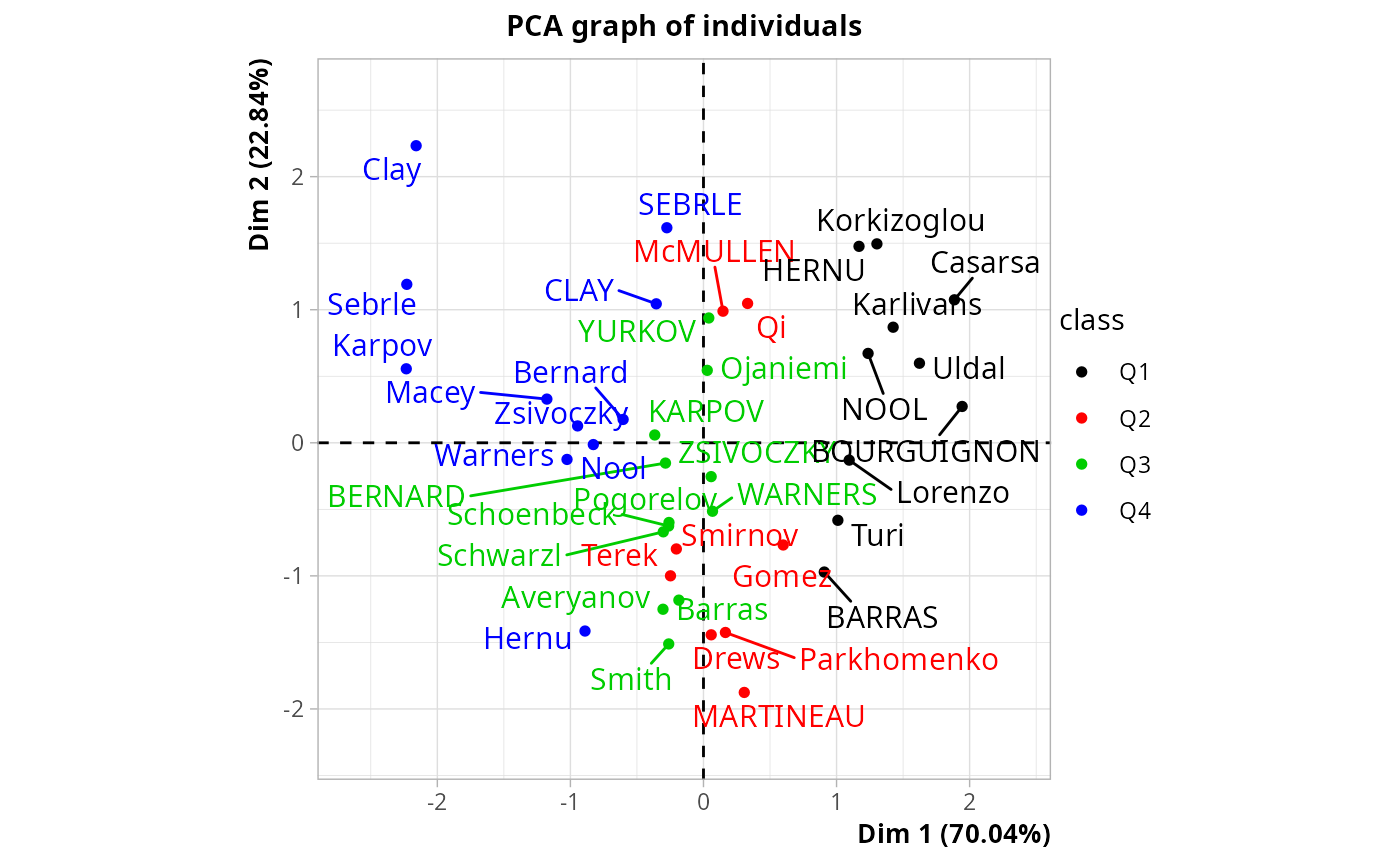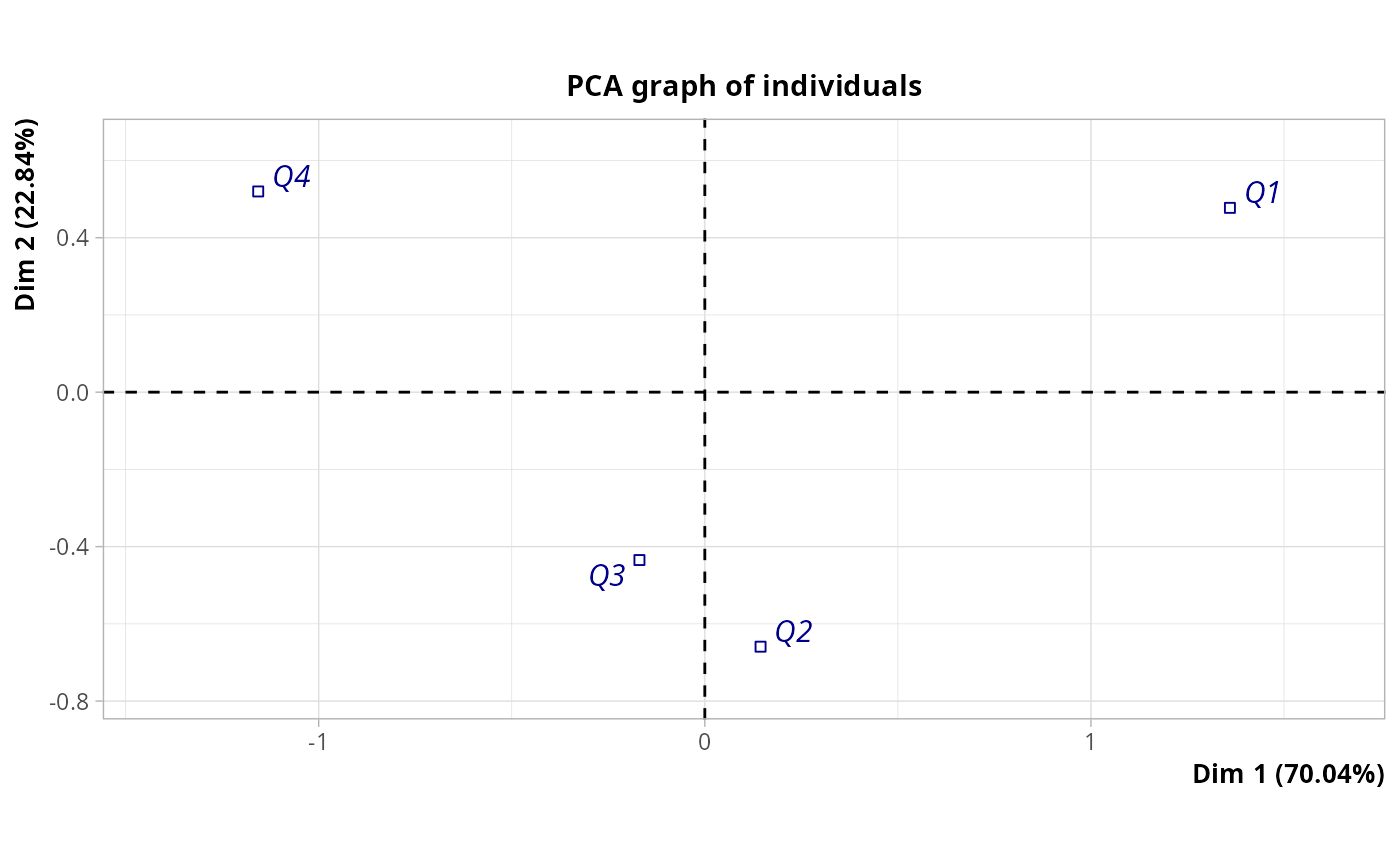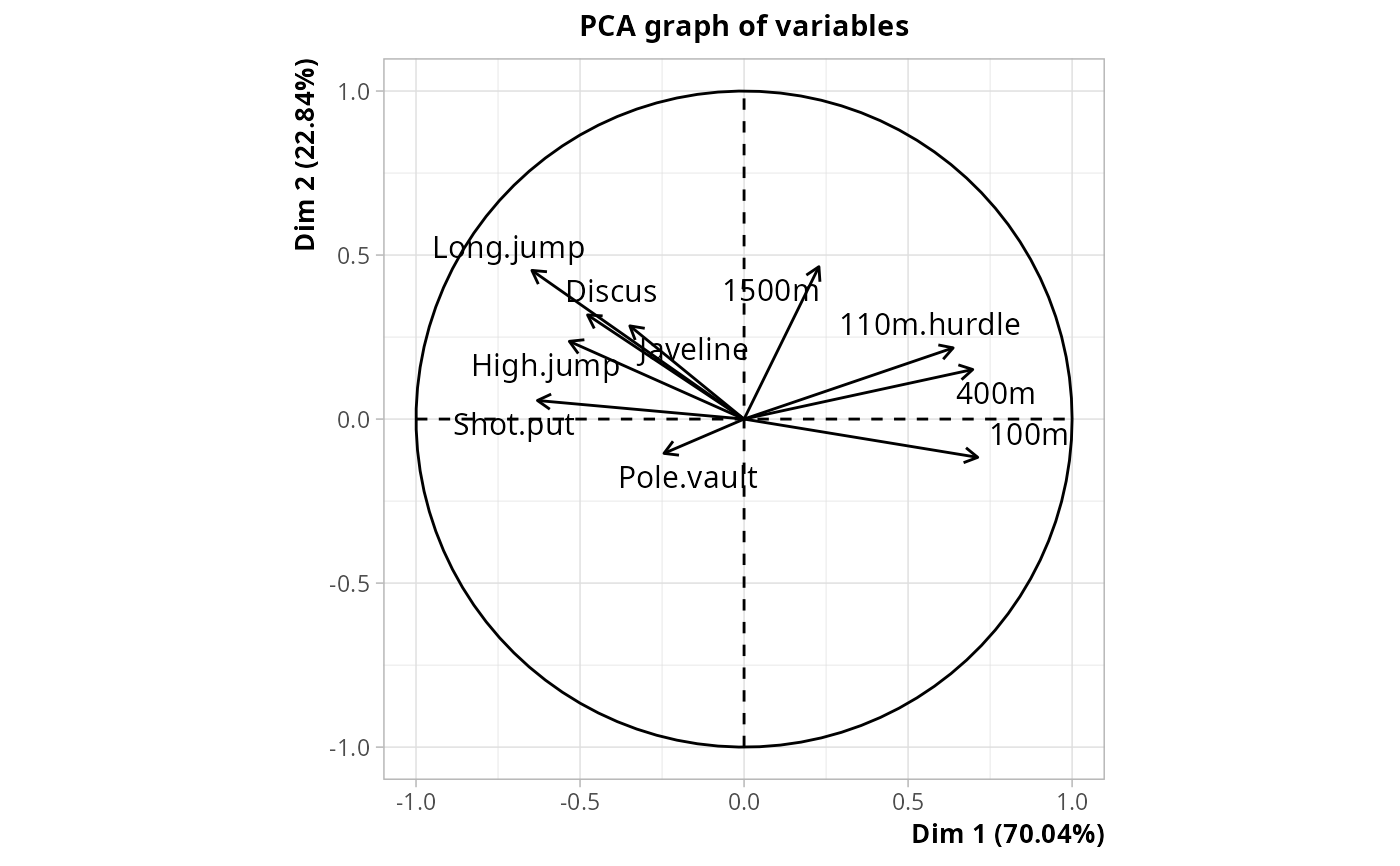Descriptive discriminant analysis, aka "Analyse Factorielle Discriminante" for the French school of multivariate data analysis.

DA(data, class, row.w = NULL, type = "FR")

## Arguments

data

data frame with only numeric variables

class

factor specifying the class

row.w

numeric vector of row weights. If NULL (default), a vector of 1 for uniform row weights is used.

type

If "FR" (default), the inverse of the total covariance matrix is used as metric. If "GB", it is the inverse of the within-class covariance matrix (Mahalanobis metric), which makes the results equivalent to linear discriminant analysis as implemented in lda function in MASS package.

## Details

The results are the same with type "FR" or "GB", only the eigenvalues vary. With type="FR", these eigenvalues vary between 0 and 1 and can be interpreted as "discriminant power".

## Value

An object of class PCA from FactoMineR package, with class as qualitative supplementary variable, and one additional item :

cor_ratio

correlation ratios between class and the discriminant factors

## Author

Marie Chavent, Nicolas Robette

## Note

The code is adapted from a script from Marie Chavent. See: https://marie-chavent.perso.math.cnrs.fr/teaching/

bcPCA, PCAiv

## Examples

library(FactoMineR)
data(decathlon)
points <- cut(decathlon$Points, c(7300, 7800, 8000, 8120, 8900), c("Q1","Q2","Q3","Q4")) res <- DA(decathlon[,1:10], points) # plot of observations colored by class plot(res, choix = "ind", invisible = "quali", habillage = res$call$quali.sup$numero)# plot of class categories
plot(res, choix = "ind", invisible = "ind", col.quali = "darkblue")# plot of variables
plot(res, choix = "varcor", invisible = "none")